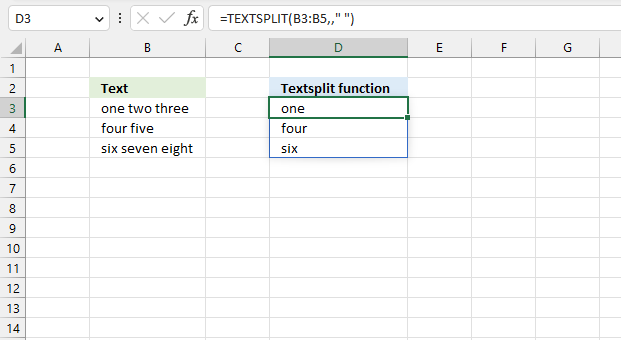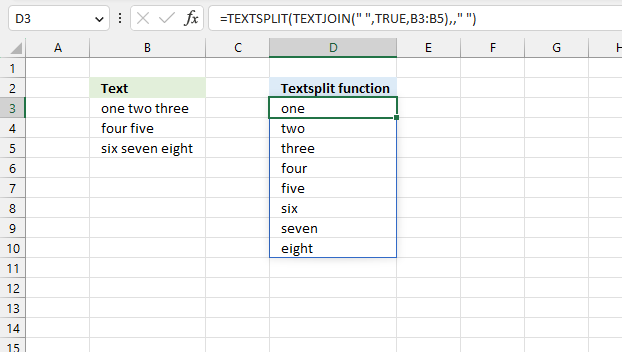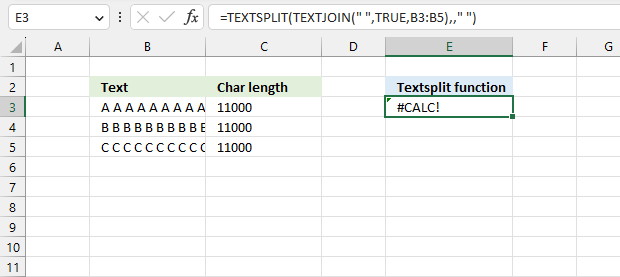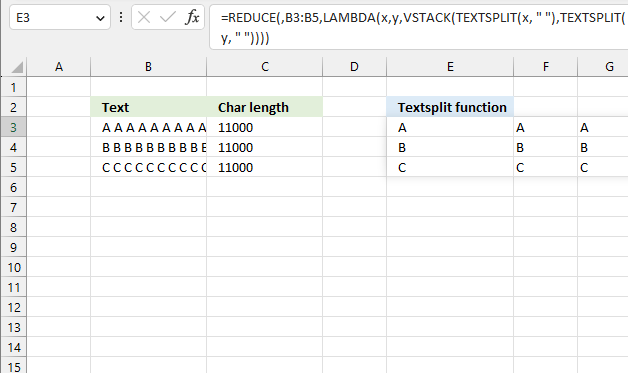Author: Oscar Cronquist Article last updated on March 08, 2023The TEXTSPLIT function splits a string into an array based on delimiting values.

Function syntax: TEXTSPLIT(Input_Text, col_delimiter, [row_delimiter], [Ignore_Empty])

The TEXTSPLIT function works only with single cells. If you try to use a cell range the TEXTSPLIT function returns only the first value in each cell. See the image above.

Formula in cell D3:

=TEXTSPLIT(B3:B5,," ")

There is a workaround for this.Join the cells using the TEXTJOIN function before splitting the strings.

Formula in cell D3:

=TEXTSPLIT(TEXTJOIN(" ",TRUE,B3:B5),," ")

This workaround is fine if the total character length is not above 32767 characters.The image above shows a scenario where the total character count exceeds 32767 characters, the formula returns #CALC! error.

There is a workaround for this as well, the Excel 365 formula below doesn't have this limitation.Excel 365 formula in cell E3:

=REDUCE(,B3:B5,LAMBDA(x,y,VSTACK(TEXTSPLIT(x, " "),TEXTSPLIT(y, " "))))

### Explaining the formula in cell E3

#### Step 1 - Split value into substrings

The TEXTSPLIT function splits a string into an array based on delimiting values.

Function syntax: TEXTSPLIT(Input_Text, col_delimiter, [row_delimiter], [Ignore_Empty])

TEXTSPLIT(y, " ")

#### Step 2 - Add arrays vertically

The VSTACK function combines cell ranges or arrays. Joins data to the first blank cell at the bottom of a cell range or array (vertical stacking)

Function syntax: VSTACK(array1,[array2],...)

VSTACK(TEXTSPLIT(x, " "),TEXTSPLIT(y, " "))

#### Step 3 - Create a LAMBDA function

The LAMBDA function build custom functions without VBA, macros or javascript.

Function syntax: LAMBDA([parameter1, parameter2, …,] calculation)

LAMBDA(x,y,VSTACK(TEXTSPLIT(x, " "),TEXTSPLIT(y, " ")))

#### Step 4 - Pass each cell value in cell range B3:B5 to the LAMBDA function

The REDUCE function applies each value in a cell range or array to a LAMBDA function, the result is a total value.

In this example, it is the opposite, each cell value is split into multiple substrings and the VSTACK function adds the arrays. The result is a larger array than the original size.

REDUCE(,B3:B5,LAMBDA(x,y,VSTACK(TEXTSPLIT(x, " "),TEXTSPLIT(y, " "))))

returns

{"A","A","A", ... ,"A";
"B","B","B", ... ,"B";
"C","C","C", ... ,"C"}

### Get the Excel file